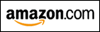MIXED INTEGRATION QUIZ #1

This web exercise gives practice with a wide variety of indefinite integration problems.
(A few definite integrals are thrown in, too.)
All the calculus steps are shown in the solutions, but only minimal algebraic simplication is done.

In this exercise, all functions are assumed to have the required properties for a particular situation.
For example, any function in a denominator is assumed to be nonzero, any function inside a logarithm is assumed to be positive, and so on.

In general, integration is more difficult than differentiation.
The following list of questions offers a thought process to help you come up with an appropriate tool for handling a given integration problem:

## IS IT JUST A DIFFERENTIATION RULE, IN REVERSE?

The first question you should always consider is:

Do I know a function whose derivative is blah?
Is this just a differentiation formula, ‘in reverse’?

### EXAMPLE:

$\displaystyle\int \sec\, x\,\tan\, x\ dx =\ ?$

Do you know a function whose derivative is $\,\sec\,x\,\tan\,x\,$?
Yes! Recall that $\frac{d}{dx} \sec\,x = \sec\, x\,\tan x\,$; so, $\,\sec x\,$ is an antiderivative of $\,\sec\, x\, \tan\, x\,$.
Thus: $\displaystyle\int \sec\, x\,\tan\, x\ dx = \sec\,x + C$

### EXAMPLE:

$\displaystyle\int \frac{1}{\sqrt{1-x^2}}\,dx =\ ?$

Do you know a function whose derivative is $\,\frac{1}{\sqrt{1-x^2}}\,$?
Yes! Recall that $\frac{d}{dx} \arcsin\,x = \frac{1}{\sqrt{1-x^2}}\,$; so, $\,\arcsin x\,$ is an antiderivative of $\,\frac{1}{\sqrt{1-x^2}}\,$.
Thus: $\displaystyle\int \frac{1}{\sqrt{1-x^2}}\,dx =\ \arcsin\,x + C$
Master the ideas from this section
by practicing the exercise at the bottom of this page.

On this exercise, you will not key in your answer.
However, you can check to see if your answer is correct.Is this web exercise helping you? I'm spending many hours creating it. Please help me to help you... just watch my 15-second video to see how easy it is to support my work, at absolutely no cost to you! (Really!) Thank you!